Numerical Checks
for Multiple Choice Questions

The graphing calculator allows for several methods of checking or comparing multiple choice answers.  Let's look at the checking of algebraic problems using a numerical format.

Process:

 Choose your "favorite" positive one-digit (for ease) integer value and store the value in x (do not pick 0 or 1).   To store a 7:   7 STO (key) x Hit ENTER.  Enter the algebraic expression from the problem on the home screen using x as your variable.
 Hit ENTER to get your numerical value. Enter each of the possible multiple choice answers on the home screen. Hit ENTER to get their numerical values. Find the answer that matches the answer from the problem.1. The product of 2x3 and 6x5 is      (1) 10x8       (2) 12x8       (3) 10x15      (4) 12x15 The number 7 was chosen as the "favorite" number.  Remember "product" means to multiply.  The expression was evaluated and the answer was 69177612.  The multiple choice answers were then checked to see which yields the same answer.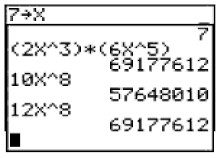2.  The sum of 3x2 + 4x - 2 and x2 - 5x + 3 is       (1) 4x2 + x - 1       (2) 4x2 - x + 1       (3) 4x2 + x + 1       (4) 4x2 - x  - 1 The number 7 was chosen.  The sum was entered.  ENTER was hit.  The multiple choice answers were entered until the matching answer was found.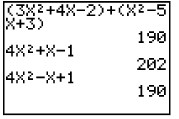3.  Express as a single fraction: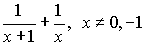(1)(2)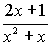(3)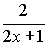(4)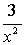The number 7 was stored in x.  The expression was carefully entered being sure to use parentheses for the first denominator.  ENTER was hit.  The multiple choice answers were entered, again being careful to use parentheses for the fraction's numerators and denominators.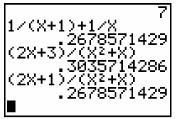Finding Your Way Around TABLE of  CONTENTS